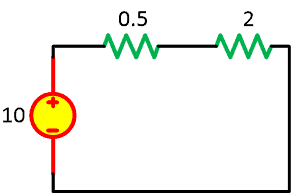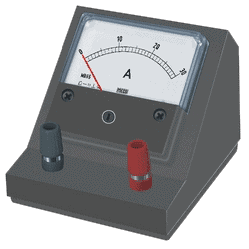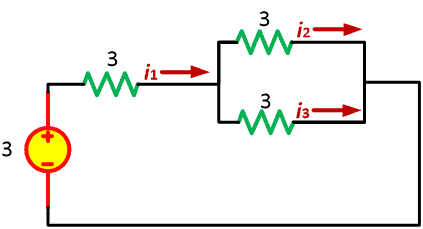# How and What Does an Ammeter MeasureThe three essential measurement tools for electrical things: voltmeters, ammeters, and ohmmeters. You must have used these tools, not one or two of them, but all of them. There is no way an electrical engineer has no experience in those things. They are basically easy to operate and to be made, but for now, let us focus on ammeters. For that reason, we will learn what does an ammeter measure for now.

An amperemeter is a device to measure the current in an electric circuit or more precise, the flow of electricity. Like what we have read in the basic explanation of an electric circuit, the measurement unit of electric current in Amperes, with the symbol of “A”.

Contents

Hence, the ammeter or amperemeter is a tool to measure how much “Ampere” in the circuit. You don’t have to get surprised if you find the “ampmeter” because people often have mistaken it.

It sounds quite similar but the right one is “ammeter”. Weird? But it is what it is. It’s not that seriously wrong but sounds so weird.

## What is Ammeter

The electric current has the SI unit of Ampere, hence its measuring tool is named Amperemeter or simply Ammeter. Even though there are two types of current: Alternating Current and Direct Current, the ammeter has no problem with measuring both.

From this short explanation we conclude from the question:

What Does an Ammeter Measure?
The ammeter is used to measure the electric current in the electric circuit, measured by Ampere (A).

The ammeter is designed using a moving coil with a pointer moved by a galvanometer. Don’t mistake it with a voltmeter when connecting it with an electric circuit. You should connect the ammeter in series with the circuit element. An ammeter is designed to have a very low resistance inside it.

Why?

Using Ohm’s Law where I = V/R is obvious that we need the resistance to be as low as possible because we don’t want to change the current value. Imagine the scheme below, the circuit has a 10-V voltage source and 2-Ω resistor. We will add a 0.5-Ω resistor as the ammeter’s resistance.Even if the ammeter has 0.5, it will still affect the current in the circuit. The current inside the circuit is supposed to be 10/2 = 5 A. The “0.5 resistance” ammeter will make the current goes down to 10/2.5 = 4 A.

It is a waste of course.

Now you understand why the ammeter is designed to have very small resistance as close as to zero.

To not affect the current value, the ammeter uses a small resistor connected in parallel with the galvanometer. The purpose of this design is to make all the current flows through the resistor.

Why?

Just as you learned in basic electronics, more current will flow through a lesser resistance branch.

The next problem: is ammeter a digital measuring tool? The answer is NO. An ammeter is an analog tool. You may find or use the “digital” ammeter, but it doesn’t mean the ammeter is operated in a digital manner. The ammeter is not even mechanical. The digital ammeter you use must have a digital display (7-segment display), but it is because of the converter.

The digital multimeter uses an ADC (Analog-to-Digital Converter) provided by a microcontroller that does all the calculation and the display through a resistor.

Ideally, an ammeter has zero resistance so the ammeter will not change any value in the circuit. But as we realize already, the ideal condition is only occurred in mathematical analysis, not in practical analysis. Even a conductor wire has a very small resistance.

You need to be careful when using the ammeter. Like stated above, the ammeter needs to be connected in series with the branch. If you connect the ammeter in parallel, the current will be very high (can be assumed “short-circuited”) and blow the fuse, destroy the ammeter, or even break the circuit components.

## Ammeter Function

### Galvanometer and Ammeter

A galvanometer is able to detect the value and direction of the current in a circuit. Just as stated above, it has a pointer attached to the armature, made from coils. The display is calibrated to read its movement results.

So what is the difference between the galvanometer and ammeter?

If you have seen the most basic dc circuit then you realize that the armature can be moved by a set of magnets while the armature is energized by electric current. The same concept can be used to differentiate between galvanometer and ammeter:

The galvanometer needs a set of magnets, while the ammeter doesn’t need one.

The other difference is the galvanometer is only capable of measuring DC.

You can imagine it right? Why can’t it measure ac value? Since the AC has negative polarity, it will move the pointer in the opposite direction. Pretty messed up in my opinion.

So how does the ammeter measure AC? While the DC ammeter still uses the moving coil and magnet principle, the AC ammeter counts the iron pieces which are moved in the presence of the electromagnetic force of fixed coil wire.

The ammeter symbol for both AC and DC is still the same. Just like a voltmeter but we use the letter ‘A’ instead. You may find it in the next section, how do we use an ammeter.

### Shunt Resistance

There are two characteristics of a galvanometer:

• A very sensitive device even for a small change of electric current.
• Can’t measure high electric potential.

Since we should not change the electric current, we are only permitted to use a very small resistance. But how do we do it with a galvanometer?

We connect the resistor in a parallel with the galvanometer. Since it is a “parallel” connection, we may call it a shunt resistance. (Shunt = Parallel)

Remember what we have talked about above, the reason we put the ammeter in a series connection with the circuit? We will use the shunt resistance to draw all the current through it so that the galvanometer will only receive a very small current.

This way the galvanometer can measure much higher current. Of course, the shunt resistance will also protect the galvanometer at the same time.

How do we determine the value of the shunt resistance? Observe the equation below:Where:

S = shunt resistance
G = resistance of the galvanometer
Ig = maximum current that can be passed through the galvanometer for full-scale deflection
I = the current to be measured

Because I is the current we measure, then the Ig is the only current permitted to pass through the galvanometer for full-scale deflection. And the rest current (IIg) have to flow through the shunt resistance.

We consider the G and S in parallel.

The effective resistance of the ammeter is expressed as:## How Does an Ammeter Work

The ammeter is designed to measure the electric current in a circuit.

How does it work?

The ammeter will measure the current flowing through a set of coils with very low resistance and inductive reactance. The impedance needs to be kept in a very small number so that the ammeter won’t change the current value because of its extra impedance.The image above is a moving-coil ammeter and we often call it an analog ammeter. Inside it, there are fixed magnets that are designed to oppose the electric current flowing through it. Its indicator pointer is moved by an armature, placed in the center of the magnet (similar to the simple dc motors). The pointer is positioned in a fine place with the scale and number in the display screen.

The most important thing of any measurement tool is they shouldn’t change the variable values in the circuit. The voltmeter, ammeter, and ohmmeter are prohibited to change the voltage, current, and resistance inside the circuit.

## How and What Does an Ammeter Measure

After learning what ammeter and galvanometer, let us put them into practice: how and what does an ammeter measure.

What we are supposed to understand here are:

• Understanding what does an ammeter measure
• Knowing how to use an ammeter to measure current

### Understanding what does an ammeter measure

If you are reading this post I bet you have understood what is current. It is hard to learn an ammeter if you don’t even know what an ammeter measure is. Everything you need to read first can be accessed in my post about what are electric currents. Summary,

Electric current is the change of charge in a period of time, measured in amperes (A) and a charge is an atomic particle in an electrical system, measured in coulombs

Don’t forget to connect the ammeter in series with the circuit. If you mistakenly connect it in parallel, it will act as a short-circuit.

### Knowing how to use an Ammeter to measure current

For example, let us use a simple electric circuit below. We will use a 3V voltage source and a set of 3 resistors with 10-Ω resistance. From Ohm’s law, we will easily know that the current will be 1 Ampere. Since it is easy to calculate a circuit which is very simple, we don’t really need an ammeter.

But what will we do if the circuit is a complex one, with a lot of components and a mix of the series-parallel connection while we have no luxury of time? The measuring tools take place here.

Anyway, let us analyze the circuit below:Let’s calculate the i1, i2, and i3.

For a beginning, we will find the currents with basic Ohm’s law first to validate the measurement tools reading later.

For i1, since it is the current in series with a voltage source, we can assume it as total current in the circuit. To calculate the total current in the circuit, we need to calculate the total resistance in the circuit first.And then the total resistanceThe total current isFor i2 and i3 we can use current division. Since R2 and R3 have the same resistance, we will split the total current by 2. Hence,

i2 = 0.1A and i3 = 0.1 A

We have the current values here. Time to use a different approach with an ammeter.

How much resistance does an ammeter have? Let’s assume it has 0.01-Ω.

For i1, we will put an ammeter between the voltage source and R1. the circuit become:Like what you have noticed, the i1 is 0.19998 A.It is very close to the 2 A if we use Ohm’s law. Why are they different? Because if we use mathematical methods, we assume that every component is in ideal condition. The ideal ammeter has zero inner resistance which is impossible in a practical way. The ammeter’s resistance of 0.01-Ω reduces the total current a little bit and we can ignore the difference.

Moving on to the i2 and i3,We get 0.09999 A for i2 and i3 instead of 0.1 A. And again, we can ignore the differences.

### How does ammeter measure current?

The ammeter will measure the current flowing through a set of coils with very low resistance and inductive reactance. The impedance needs to be kept in a very small number so that the ammeter won’t change the current value because of its extra impedance.

### What do ammeters and voltmeters measure?

An ammeter is used to measure electric current while a voltmeter is used to measure electric voltage.

### What is the principle of ammeter?

The impedance needs to be kept in a very small number so that the ammeter won’t change the current value because of its extra impedance.

### Do ammeters have high resistance?

The resistance needs to be kept in a very small number so that the ammeter won’t change the current value because of its extra resistance.